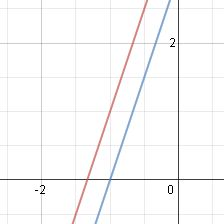# Graphs of Parallel and Perpendicular Lines in Linear Equations

An error occurred trying to load this video.

Try refreshing the page, or contact customer support.

Coming up next: How to Write a Linear Equation

### You're on a roll. Keep up the good work!

Replay
Your next lesson will play in 10 seconds
• 0:02 Linear Equations
• 1:17 Parallel Equations
• 2:10 Perpendicular Equations
• 3:53 A Graph
• 5:07 Lesson Summary
Save Save

Want to watch this again later?

Timeline
Autoplay
Autoplay
Speed Speed

#### Recommended Lessons and Courses for You

Lesson Transcript
Instructor: Yuanxin (Amy) Yang Alcocer

Amy has a master's degree in secondary education and has taught math at a public charter high school.

When we deal with linear equations, we sometimes come across equations that are parallel to each other or perpendicular to each other. You will see what they look like when graphed in this video lesson.

## Linear Equations

Linear equations, as the name suggests, are equations of straight lines. When you graph these types of equations, you get a straight line. Now these lines can be horizontal, vertical, or slanted. When you pair two of these equations together, you get to compare them to each other. Sometimes the equations intersect each other, other times they won't, and yet other times they may form a perfectly perpendicular intersection where each of the angles at the intersection is 90 degrees.

In this video lesson, it is the graphs of a pair of linear equations that we are considering. We will consider the case where the lines never meet (when they are parallel) and the case where the lines form a perfectly perpendicular intersection. We will see the interesting properties that each case presents. You will see how these properties actually make it easier for you to identify your equations that are parallel and your equations that are perpendicular.

We begin by recalling that our linear equations are most often written in slope-intercept form, which is y = mx + b, where m is our slope and b is our y-intercept. It is this form that will be most useful for us in determining whether two lines are parallel or perpendicular. Let's see how.

## Parallel Equations

Parallel equations are equations where the lines never meet. Picture two roads that run side by side and you will have a pair of parallel lines. These roads are parallel because they never meet or cross each other. When we have a pair of parallel lines and their equations, we can easily tell that they are parallel just by comparing the equations.

You see, when two lines are parallel, their slopes will be the same and their y-intercepts will be different. So, what we are looking for is the same slope with different y-intercepts. For example, the equations y = 3x + 4 and y = 3x + 3 are parallel because their slopes are the same and their y-intercepts are different. What happens when we graph them? We get two lines that never intersect like this:## Perpendicular Equations

Now, what about perpendicular lines, lines that form 90 degree angles when they intersect? Picture the intersections in downtown areas of a city, and you are looking at perpendicular roads. What do we look for in this situation?

In this case, the only thing that we need to look at is the slope. Perpendicular lines have slopes that are negative reciprocals of each other. What does this mean? It means that we take one slope and divide 1 by that slope, and then we put a negative sign in front.

So, if one slope is 3, then the negative reciprocal is -1/3. The negative reciprocal of -1/3 is -(1 / (-1/3)), which becomes 3 after we evaluate it applying our basic algebra skills. So, we can say that 3 and -1/3 are negative reciprocals of each other.

Another way that we can think of negative reciprocals is that they are negative flipped versions of each other. Notice how the numerator and denominators have changed sides and we have a negative sign. So, if we see a pair of equations whose slopes are negative reciprocals of each other, then we are looking at a pair of perpendicular lines.

To unlock this lesson you must be a Study.com Member.

### Register to view this lesson

Are you a student or a teacher?

#### See for yourself why 30 million people use Study.com

##### Become a Study.com member and start learning now.
Back
What teachers are saying about Study.com

### Earning College Credit

Did you know… We have over 200 college courses that prepare you to earn credit by exam that is accepted by over 1,500 colleges and universities. You can test out of the first two years of college and save thousands off your degree. Anyone can earn credit-by-exam regardless of age or education level.